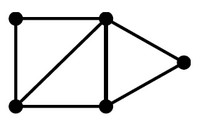# Graph - Degree

In a graph, the degree of a vertex (node) is the number of edges that touch the node (vertex) (ie the number of adjacent edges)

degree is also known as valency

## Documentation / Reference

Recommended PagesGraph - (Node | Vertice | Vertex | Point)

A node in a graph model an entity The model graph contains connected the nodes: containing any number of attributes (key-value-pairs). tagged with labels (to label, attach metadata—​index or...Graph - Operator (Operations)

on a graph Operations Description degree(G, x) the number of adjacent (connected) edge for the node x (ie degree) adjacent(G, x, y) adjacent tests whether there is an edge from the vertex x...